## Selina Concise Mathematics Class 10 ICSE Solutions Chapter 13 Section and Mid-Point Formula Ex 13A

These Solutions are part of Selina Concise Mathematics Class 10 ICSE Solutions. Here we have given Selina Concise Mathematics Class 10 ICSE Solutions Chapter 13 Section and Mid-Point Formula Ex 13A.

Other Exercises

Question 1.
Calculate the co-ordinates-of the point P which divides the line segment joining:
(i) A (1, 3) and B (5, 9) in the ratio 1 : 2
(ii) A (-4, 6) and B (3, -5) in the ratio 3 : 2
Solution:
(i) Let co-ordinates of P be (x,y)Question 2.
In what ratio is the line joining (2, -3) and (5, 6) divided by the x-axis ?
Solution:
Let the point P (x, 0) divides in the ratio of m1 : m2 line joining the points A (2, -3) and B (5, 6)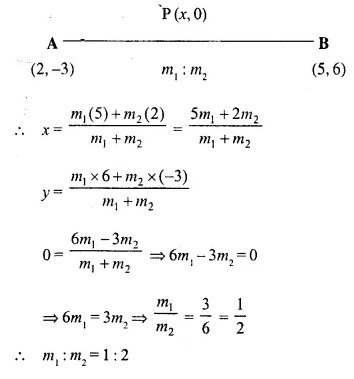Question 3.
In what ratio is the line joining (2, -4) and (-3, 6) divided by the y-axis ?
Solution:
Let the point P (0, y) divides the line joining the points A (2, -4) and (-3, 6) in the ratio of m1 : m2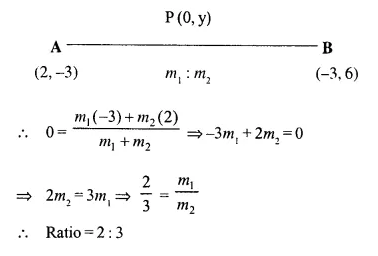Question 4.
In what ratio does the point (1, a) divide the join of (-1, 4) and (4, -1)? Also, find the value of ‘a’.
Solution:
Let the point P (1, a) divides the line joining the points (-1, 4) and (4, -1) in the ratio of m1 : m2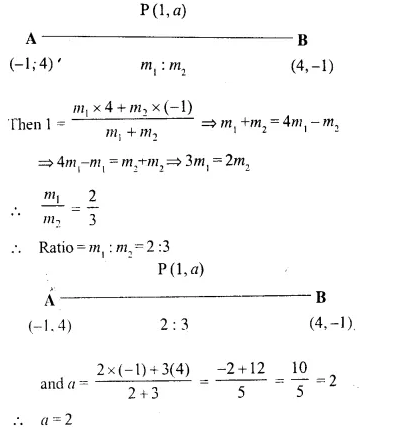Question 5.
In what ratio does the point (a, 6) divide the join of (-4, 3) and (2, 8) ? Also, find the value of ‘a’.
Solution:
Let the point P (a, 6) divides the line joining the points A (-4, 3), B (2, 8) in the ratio of m1 : m2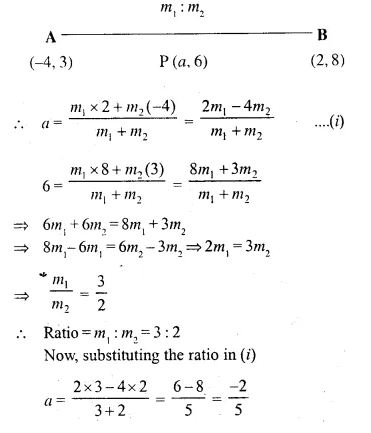Question 6.
In what ratio is the join of (4, 3) and (2, -6) divided by the x-axis. Also, find the co-ordinates of the point of intersection.
Solution: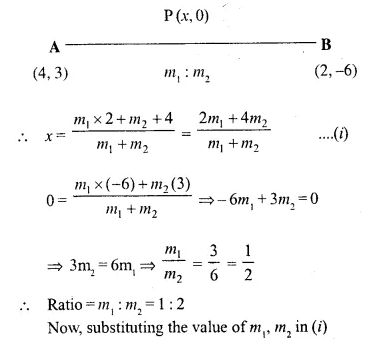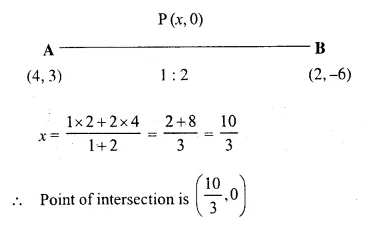Question 7.
Find the ratio in which the join of (-4, 7) and (3, 0) is divided by the y-axis. Also, find the co-ordinates of the point of intersection.
Solution:
Let, the points (0, y) be the point of intersection which divides the line joining the points A (-4, 7) and B (3, 0)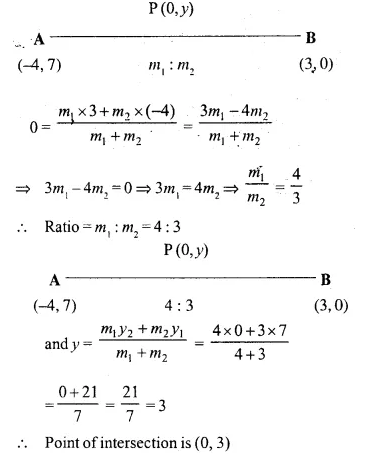Question 8.
Points A, B, C and D divide the line segment joining the point (5, -10) and the origin in five equal parts. Find the co-ordinates of A, B, C and D.
Solution:
Points A, B, C and D divide the line segment joining the points (5, -10) and origin (0, 0) in five equal parts
Let co-ordinates of A be (x, y) which divides PO in the ratio of 1 : 4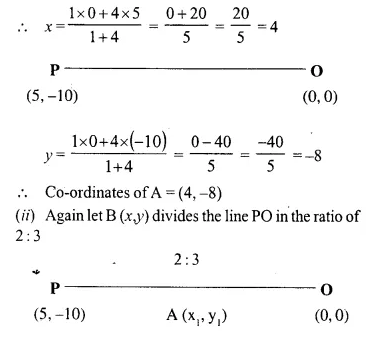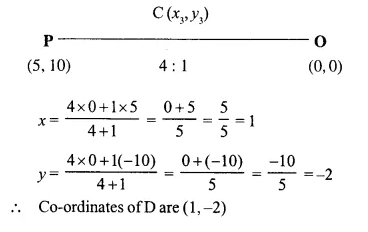Question 9.
The line joining the points A (-3, -10) and B (-2, 6) is divided by the point P such that = $$\frac { PA }{ PB }$$ = $$\frac { 1 }{ 5 }$$, find the co-ordinates of P.
Solution:
Let the co-ordinates of P be (x, y) which divides the line joining the points A (-3,-10) and B (-2,6) in the ratio of AP : PB i.e. (5 – 1) : 1 or 4 : 1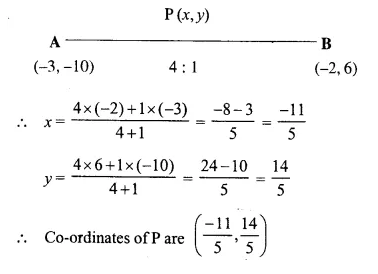Question 10.
P is a point on the line joining A (4, 3) and B (-2, 6) such that 5AP = 2BP. Find the co-ordinates of P.
Solution: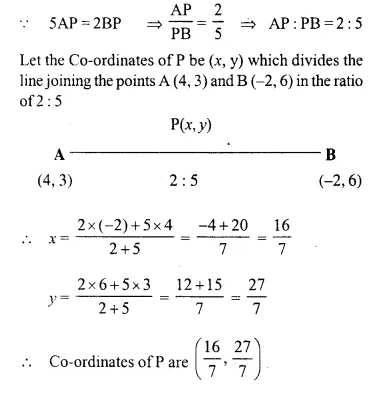Question 11.
Calculate the ratio in which the line joining the points (-3, -1) and (5, 7) is divided by the line x = 2. Also, find the co-ordinates of the point of intersection.
Solution:
Let the point P (2, y) divides the line joining the points A (-3, -1) and B (5, 7) in the ratio of m1 : m2Question 12.
Calculate the ratio in which the line joining A (6, 5) and B (4, -3) is divided by the line y = 2.
Solution:
Let the point P (x, 2) divides the line joining the points A (6, 5) and B (4, -3) in the ratio of m1 : m2Question 13.
The point P(5, -4) divides the line segment AB, as shown in the figure, in the ratio 2 : 5. Find the co-ordinates of points A and B.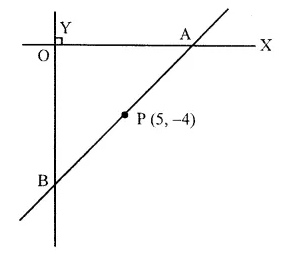Solution:
From the figure, the line AB intersects x-axis at A and y-axis at B.
Let the co-ordinates of A (x, 0) and B (0, y) and P (5, -4) divides it in the ratio of 2 : 5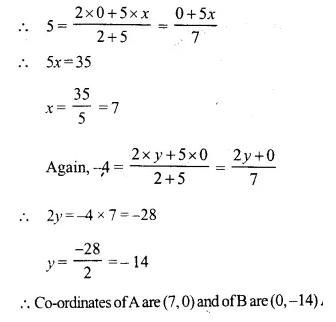Question 14.
Find the co-ordinates of the points of trisection of the line joining the points (-3, 0) and (6, 6).
Solution: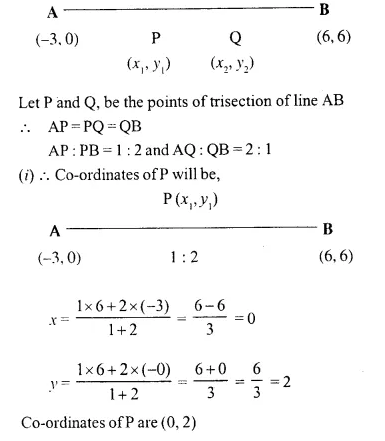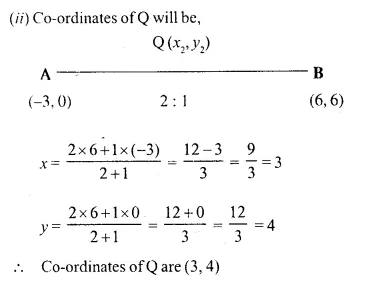Question 15.
Show that the line segment joining the points (-5, 8) and (10, -4) is trisected by the co-ordinate axes.
Solution: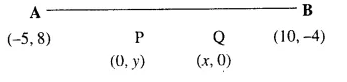Let the points A (-5, 8) and B (10, -4).
Let P and Q be the two points on the axis which trisect the line joining the points A and B.
AP = PQ = QB
AP : PB = 1 : 2 and AQ : QB = 2 : 1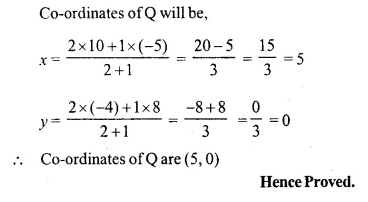Question 16.
Show that A (3, -2) is a point of trisection of the line-segment joining the points (2, 1) and (5, -8). Also, find the co-ordinates of the other point of trisection.
Solution: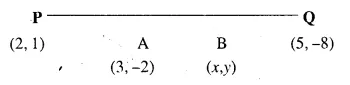Let A and B are the points of trisection of the line segment joining the points P (2, 1) and Q (5, -8), then
PA = AB = BQ.
PA : AQ = 1 : 2 and PB : BQ = 2 : 1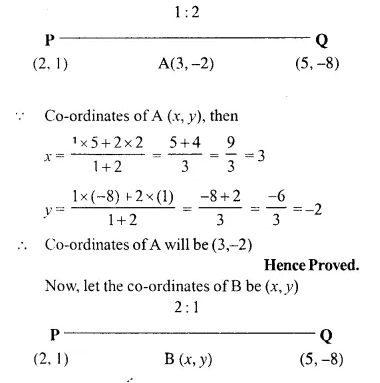Question 17.
If A = (-4, 3) and B = (8, -6)
(i) find the length of AB
(ii) In what ratio is the line joining A and B, divided by the x-axis ?
Solution: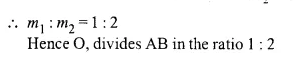Question 18.
The line segment joining the points M (5, 7) and N (-3, 2) is intersected by the y-axis at point L. Write down the abscissa of L. Hence, find the ratio in which L divides MN. Also, find the co-ordinates of L.
Solution: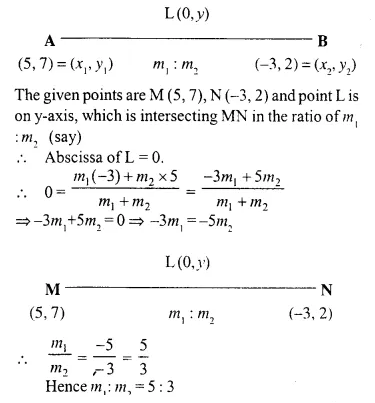Question 19.
A (2, 5), B (-1, 2) and C (5, 8) are the co-ordinates of the vertices of the triangle ABC. Points P and Q lie on AB and AC respectively, such that:
AP : PB = AQ : QC = 1 : 2.
(i) Calculate the co-ordinates of P and Q.
(ii) Show that PQ = $$\frac { 1 }{ 3 }$$ BC.
Solution: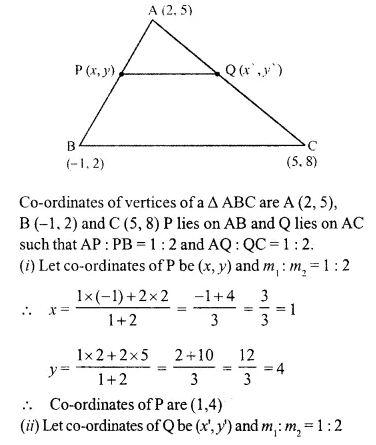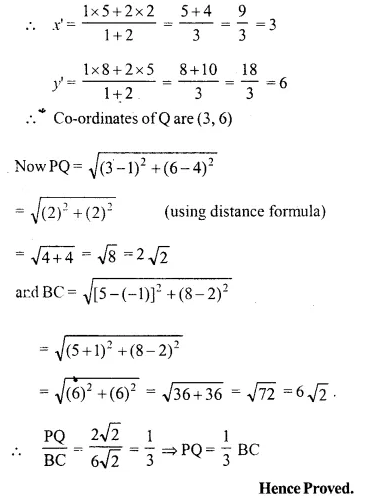Question 20.
A (-3, 4), B ( 3, -1) and C (-2, 4) are the vertices of a triangle ABC. Find the length of line segment AP, where point P lies inside BC, such that BP: PC = 2 : 3.
Solution: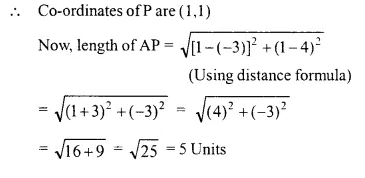Question 21.
ThelinesegmentjoiningA(2, 3)andB(6, -5) is intercepted by x-axis at the point K. Write down the ordinate of the point K. Hence, find the ratio in which K divides AB. Also, find the co-ordinates of the pointK. [1990, 2006]
Solution:
Let the line segment Intersect the x-axis at the point P
Co-ordinates of P are (x, 0)
Let P divide the line segment in the ratio K : 1 then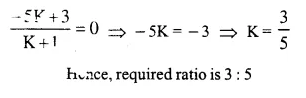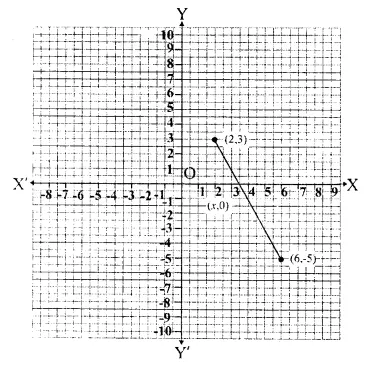Question 22.
The line segment joining A (4, 7) and B (-6, -2) is intercepted by the y-axis at the point K. Write down the abscissa of the point K. Hence, find the ratio in which K divides AB. Also, find the co-ordinates of the point K.
Solution:
Points A (4, -7), B (-6, -2) are joined which intersects y-axis at K. abscissa of K will be 0Let the coordinates of K be (0, y) and K divides AB line segment in the ratio m1 : m2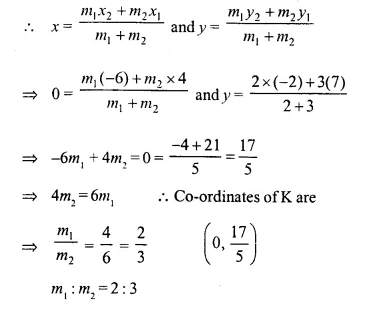Question 23.
The line joining P (-4, 5) and Q (3, 2), intersects they axis at point R. PM and QN are perpendiculars from P and Q on the x-axis. Find:
(i) The ratio PR: RQ.
(ii) The co-ordinates of R.
(iii) The areas of the quadrilateral PMNQ. 
Solution:
(i) Let divides the line joining the points P (-4, 5) and Q (3, 2) in the ratio k : 1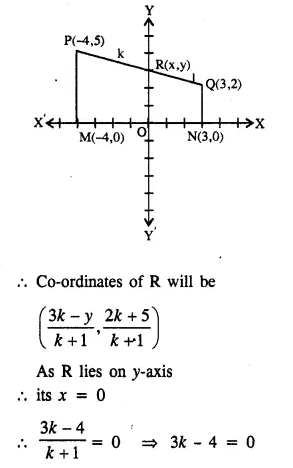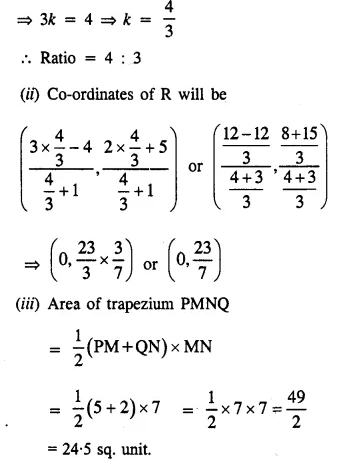Question 24.
In the given figure, line APB meets the x- axis at point A and y-axis at point B. P is the point (-4, 2) and AP : PB = 1 : 2. Find the co-ordinates of A and B.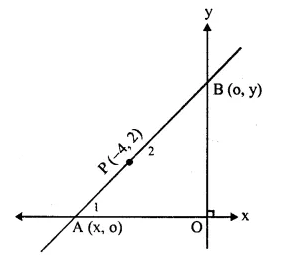Solution:
Let the co-ordinates of A be (x1, 0) (as it lies on x-axis)
and co-ordinates of B be (0, y2)
and co-ordinates of P are (-4, 2)
AP : PB = 1 : 2 i.e. m1 = 1, m2 = 2
Now, P divides AB in the ratio m1 : m2 or 1 : 2Question 25.
Given a line segment AB joining the points A (-4, 6) and B (8, -3). Find:
(i) the ratio in which AB is divided by the y-axis.
(ii) find the coordinates of the point of intersection.
(iii) the length of AB.
Solution:
(i) Let the y-axis divide AB in the ratio m : 1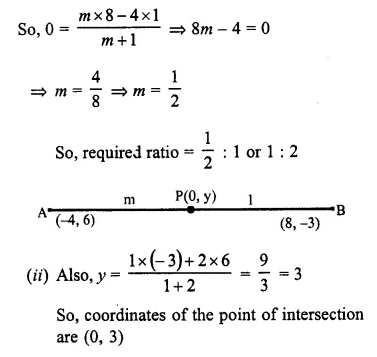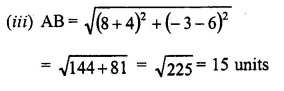Hope given Selina Concise Mathematics Class 10 ICSE Solutions Chapter 13 Section and Mid-Point Formula Ex 13A are helpful to complete your math homework.

If you have any doubts, please comment below. Learn Insta try to provide online math tutoring for you.